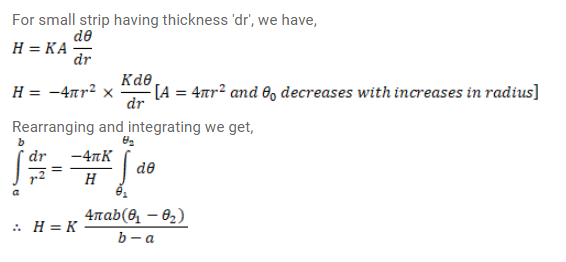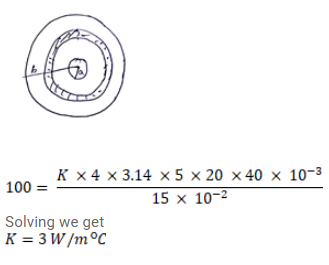# A hollow metallic sphere of radius 20cm surrounds

Question:

A hollow metallic sphere of radius $20 \mathrm{~cm}$ surrounds a concentric metallic sphere of radius $5 \mathrm{~cm}$. The space between the two spheres is filled with a nonmetallic material. The inner and outer spheres are maintained at $50^{\circ} \mathrm{C}$ and $10^{\circ} \mathrm{C}$ respectively and it is found that $100 \mathrm{~J}$ of heat passes from the inner sphere to the outer sphere per second. Find the thermal conductivity of the material between the spheres.

Solution: